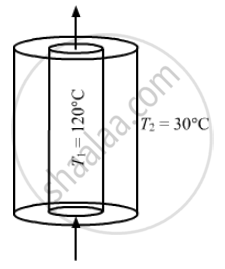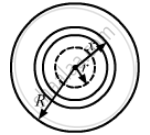Share

# Steam at 120°C is Continuously Passed Through a 50 Cm Long Rubber Tube of Inner and Outer Radii 1.0 Cm and 1.2 Cm. the Room Temperature is 30°C. Calculate the Rate of Heat Flow Through the - Physics

ConceptHeat Transfer Conduction

#### Question

Steam at 120°C is continuously passed through a 50 cm long rubber tube of inner and outer radii 1.0 cm and 1.2 cm. The room temperature is 30°C. Calculate the rate of heat flow through the walls of the tube. Thermal conductivity of rubber = 0.15 J s−1 m−1°C−1.

#### SolutionInner radii = r = 1 cm = 10–2 m

Outer radii = R = 1.2 cm = 1.2 × 10–2 m

Length of the tube, l = 50 cm = 0.5 m

Thermal conductivity, k = 0.15 Js–1 m–1 °C–1

Top ViewLet us consider a cylindrical shell of length l,

Rate of flow of heat q =( dQ)/dt

(dQ)/dt = -(KADeltaT)/dx

Here , the negative sign indicates that the rate of heat flow decreases as x increases.

q=-K(2pixl).(dT)/(dx)

int_r^R dx/x = -(2piKL)/q int_{T_1}^{T_2}dT

["ln" (x)]_r^R = (2piKL)/q (T_2 -T_1)

⇒ q = (2piKL(T_1 -T_2))/( "in" (R/r)

q = (2pi xx 0.15 xx 0.5xx(90)}/{"ln"((1.2xx10^-2)/(1xx10^-2))

q = 262.9  J/s

Is there an error in this question or solution?

#### APPEARS IN

Solution Steam at 120°C is Continuously Passed Through a 50 Cm Long Rubber Tube of Inner and Outer Radii 1.0 Cm and 1.2 Cm. the Room Temperature is 30°C. Calculate the Rate of Heat Flow Through the Concept: Heat Transfer - Conduction.
S